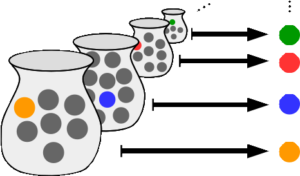# Choice Function & The Axiom of Choice Explained

A function that chooses one element out of each non-empty subset of a set A is called a choice function for A. The choice function simultaneously chooses an element from each of many sets. It isn’t a function in the calculus sense of the word (as in, one-to-one correspondence); it’s used in set theory as the underpinnings of a rather peculiar axiom called the Axiom of Choice.

For example, let’s say your non-empty subsets were the integers {1, 2, 3, …). There are an infinite amount of subsets, but for this example, let’s look at just one: A = {1, 2}. You could set your choice function to choose the largest number of each set, so it would choose 2 from this particular set. Of course, there are an infinite number of possible sets, so the choice would have to be made infinite times. You’ll end up with an infinite set of the “largest” numbers in each subset.

## The Axiom of Choice ExplainedIllustration of the axiom of choice, with each set Si represented as a jar and its elements represented as marbles. Each element xi is represented as a marble on the right. Colors are used to suggest a functional association of marbles after adopting the choice axiom. The existence of such a choice function is in general independent of ZF for collections of infinite cardinality.

The choice function is the machinery behind the Axiom of Choice, defined as (Halmos, 2017): “The Cartesian product of a non-empty family of non-empty sets is non-empty.” The axiom has many equivalent statements (Enderton, 1977). For example: “Every family of nonempty sets has a choice function” (Hill, n.d.).

The following concrete example (Rahim, 2014) illustrates how the axiom of choice works with material objects. Let’s say you have an infinite number of pairs of socks. The choice function pulls one of each pair, simultaneously. As you have an infinite number of pairs, it might be hard to wrap your head around what the set of all chosen socks looks like. And in fact, that’s one of the problems with the axiom: Although it’s intuitively true (it must be possible to choose one sock from each pair, no matter how many pairs they are), the axiom makes no mention of how the process works. Note that if the set of all pairs of socks were finite, the Axiom of Choice wouldn’t be needed.

So this presents a bit of a conundrum; Although it’s intuitively true, it can’t be proved using other axioms. This makes it quite different from other axioms. And for many years, there was doubt it should even be classified as an axiom at all.

## Choice Function: References

Axiom of choice Explained Image: Fschwarzentruber, , CC BY-SA 4.0 via Wikimedia Commons
Boyer, C. B. and Merzbacher, U. C. A History of Mathematics, 2nd ed. New York: Wiley, 1991.
Conway, J. H. and Guy, R. K. The Book of Numbers. New York: Springer-Verlag, pp. 274-276, 1996.
Enderton, Herbert B; Elements of Set Theory; Academic Press, 1977
Halmos, P. Naive Set Theory (Dover Books on Mathematics) Dover, 2017.
Hill J. https://www.untruth.org/~josh/math/AxiomofChoice.pdf
Mendelson, E. Introduction to Mathematical Logic, 4th ed. London: Chapman & Hall, 1997.
Rahim, Farighon Abdul, “Axioms of Set Theory and Equivalents of Axiom of Choice” (2014). Mathematics Undergraduate Theses. Paper

CITE THIS AS:
Stephanie Glen. "Choice Function & The Axiom of Choice Explained" From StatisticsHowTo.com: Elementary Statistics for the rest of us! https://www.statisticshowto.com/choice-function/